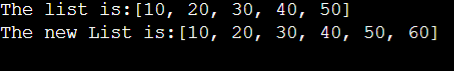New update is available. Click here to update.
Last Updated: Jun 30, 2023
Easy

## Introduction

Linked List is a linear data structure that is used to store the data. It is a dynamic data structure and can also be considered a custom data type. Linked Lists are one of the easiest Data Structures to start with and have a wide range of applications across various fields.

There is a very famous and useful method for LinkedList add() method. This method is used to add the data to our LinkedList.

There are two ways to apply this method. Let us discuss each of them:-

Recommended Topic, Floyds Algorithm And Rabin Karp Algorithm

Using the above method we can add the data at the end of the Linked List.

We need to pass the data to this method which we need to add to our LinkedList. This method appends the data at the end of the LinkedList. The above method returns true if the element is appended to the LinkedList.

Refer to the below code for more understanding.

``````import java.util.*;

public static void main(String args[]) {

System.out.println("The list is:" + list);

System.out.println("The new List is:" + list);
}
}``````

Output:Time Complexity: The time complexity for the above functions is O(1).

Space Complexity: The space complexity for the above method is O(N) to maintain all the ‘N’ elements of the LinkedList.

Using the above method we can add the data at any particular index of the LinkedList.

Syntax: boolean add(int index, Object X)

The above method returns true if at least one element is appended to the LinkedList at the given index.

Refer to the below code for more understanding.

``````import java.util.*;

public static void main(String args[]) {

System.out.println("The list is:" + list);

System.out.println("The new List is:" + list);
}
}``````

Output:Time Complexity: The time complexity for the above functions is O(N) because we need to traverse to the given index.

Space Complexity: The space complexity for the above method is O(N) to maintain all the N elements of the LinkedList.

### What is the time complexity for both functions?

The time complexity for the first function is O(1), whereas the Time complexity for the second method is O(N) because we need to traverse to the given index.

## Conclusion

In this blog, we have covered the following things:

2. Then we discussed the two types of LinkedList add methods in Java.

Recommended Problems -